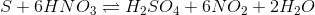# AP Chemistry : Balancing Equations

## Example Questions

← Previous 1

### Example Question #1 : Stoichiometry

Balance the following equation:

NaOH + H2SO4 → H2O + Na2SO4

4 NaOH + 2 H2SO4 → 4 H2O + 2 Na2SO4

2 NaOH + H2SO4 → H2O + Na2SO4

NaOH + H2SO4 → H2O + Na2SO4

4 NaOH + H2SO4 → 2 H2O + 2 Na2SO4

2 NaOH + H2SO4 → 2 H2O + Na2SO4

2 NaOH + H2SO4 → 2 H2O + Na2SO4

Explanation:

Balancing equations:

there is 1 NaOH and 1 H2SO4 in the reactants; and 1 H2O and 1 Na2SO4 in the products

steps:

1) balance the Na first; get 2 NaOH

2) balance H next; get 1 H2SO4, 2 H2O

3) check to make sure that O and S balance

### Example Question #1 : Balancing Chemical Equations

_Fe2O3 + _HCl ⇌ _FeCl3 + _H2O

The Following question will be based on the unbalanced reaction above

For the reaction above to be balanced what coefficient should be in front of the compound HCl?

3

1

2

4

6

6

Explanation:

Balancing reactions is best acheived by using a stepwise approach.  It is useful to work through each atom making sure it is present in a balanced fashion on both sides of the equation.  Starting with Fe, Fe2Ois the only molecule with Fe present on the left side of the equation and FeCl3 is the only molecule with Fe present on the right side of the equation.  Thus the molar ratio of Fe2Oto FeCl3 must be 1:2.  Moving on to O, the O on the left side of the equation exists as Fe2O3 and H2O on the right.  The molar ratio of Fe2Oto H2O is therefore 1:3.  Cl exists in HCl on the left and FeClon the right. Thus the molar ratio of HCl to FeCl3 must be 3:1. To ensure that these molar ratios are maintaned the balanced formula is then determined to be Fe2O3 + 6HCl -> 2FeCl3 + 3H2O

### Example Question #2 : Stoichiometry

Balance the following equation:

FeCl+ NOH→ Fe(OH)3 + NH4Cl

2 FeCl3 + 4 NOH5 → Fe(OH)3 + 6 NH4Cl

3 FeCl3 + 3 NOH5 → 2 Fe(OH)3 + 4 NH4Cl

FeCl3 + 3 NOH5 → Fe(OH)3 + 3 NH4Cl

3 FeCl3 + 3 NOH5 → 3 Fe(OH)3 + 3 NH4Cl

2 FeCl3 + 3 NOH5 → 2 Fe(OH)3 + 3 NH4Cl

FeCl3 + 3 NOH5 → Fe(OH)3 + 3 NH4Cl

Explanation:

The first thing to do is to balance the Cl (3 on the left; one on the right) ← add 3 for NH4Cl

Now, there are 3 N on the right and only one on the left; add a 3 to the NOH5

Check to see that the Fe, H, and O balance, which they do

### Example Question #1 : Stoichiometry

After the following reaction is balanced, how many moles of H2O can 4 moles of C2Hproduce?

__C2H6(s) + ___O2(g) → ___H2O(l) + ___CO2(g)

9

3

12

6

8

12

Explanation:

The reaction balances out to 2C2H6(s) + 7O2(g) → 6H2O(l) + 4CO2(g). For every  2 moles of  C2H6 you can produce 6 moles of H2O. Giving you a total production of 12 moles when you have 4 moles of C2H6.

### Example Question #5 : Balancing Equations

Consider the following reaction: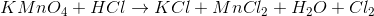When the equation is balanced, what will be the coefficient in front of HCl?

16

8

12

5

16

Explanation:

When balancing equations, the goal is to make sure that the same atoms, in both type and amount, are on both the reactant and product side of the equation. A helpful approach is to write down the number of atoms already on both sides of the unbalanced equation. This way, you can predict which compounds need to be increased on which side in order to balance the equation. It also helps to balance oxygen and hydrogen last in the equation.

In this reaction, we can balance as follows.

Reactants: 1K, 1Mn, 1Cl, 4O, 1H

Products: 1K, 1Mn, 5Cl, 1O, 2H

So, we will need to increase H2O and HCl. The final balanced equation is written below.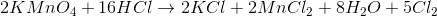### Example Question #11 : Balancing Chemical Equations

Balance the following chemical equation.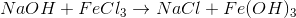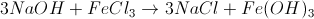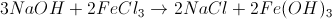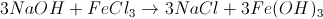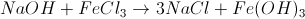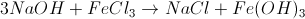Explanation:

To balance an equation, we need to make sure there is the same amount of elements to the left of the arrow as there is to the right. We also need all the charges to balance out. We notice right away that there are three chlorine atoms on the left, but only one on the right.(1Na, 1O, 1H, 1Fe, 3Cl : 1Na, 3O, 3H, 1Fe, 1Cl)

We can solve this by multiplying NaCl by three.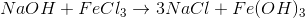(1Na, 1O, 1H, 1Fe, 3Cl : 3Na, 3O, 3H, 1Fe, 3Cl)

This causes us to have an imbalance of sodium, which we can correct by manipulating NaOH.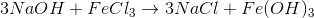(3Na, 3O, 3H, 1Fe, 3Cl : 3Na, 3O, 3H, 1Fe, 1Cl)

This is the final balanced equation. Note that it is usually easiest to manipulate oxygen and hydrogen last, since they are often involved in multiple molecules.

### Example Question #7 : Balancing Equations

Calcium hydroxide is treated with hydrochloric acid to produce water and calcium chloride. Write a balanced chemical reaction that describes this process.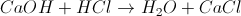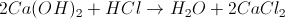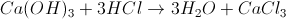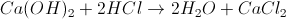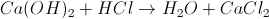Explanation:

Calcium is in the second group of the periodic table, and is therefore going to have a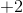oxidation number. Hydroxide ions have acharge. Calcium hydroxide will have the formula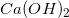.

Chloride ions have acharge and hydrogen ions have a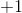charge. The formula for hydrochloric acid is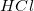.

On the products side, water has the formula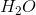and calcium chloride has the formula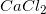.

Now that we know all of the formulas, we can write our reaction: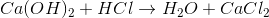In order to balance the chloride atoms, we need to add coefficients.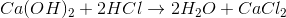### Example Question #8 : Balancing Equations

Consider the following unbalanced equation for the combustion of propane,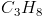: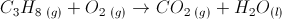If you were to combust one mole of propane, how many moles of water would you produce?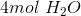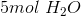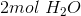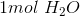Explanation:

Begin by balancing the equation. There are many ways to do this, but one method that is particularly useful is to assume that you have 1 mole of your hydrocarbon (propane), and balance the equation from there. It may be necessary to manipulate an equation further if you end up with fractions, but all you will need to do is multiply by an integer if that is the case.

First, we will balance the carbons. There are three carbons in propane, so we will make sure there are three carbons on the right side of the arrow as well: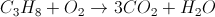This is not complete. We will next balance the hydrogens. There are eight hydrogens on the left side of the equation, so: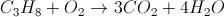The last step is to balance the oxygens on the left and right side of the equation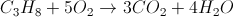Our equation is balanced and all coefficients are integers. If we begin with one mole of propane, we will produce four moles of water.

### Example Question #9 : Balancing Equations

Consider the following unbalanced equation: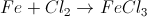How many grams of solid iron are needed to make 36.0g of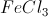? Assume that chlorine is in excess.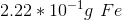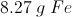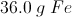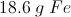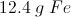Explanation:First, we will balance the equation: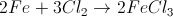Since chlorine is in excess, we know that the limiting reagent is iron.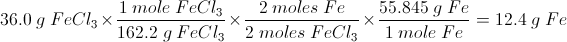### Example Question #10 : Balancing Equations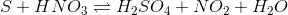In the balanced version of the preceding equation, what is the coefficient of nitrogen dioxide?